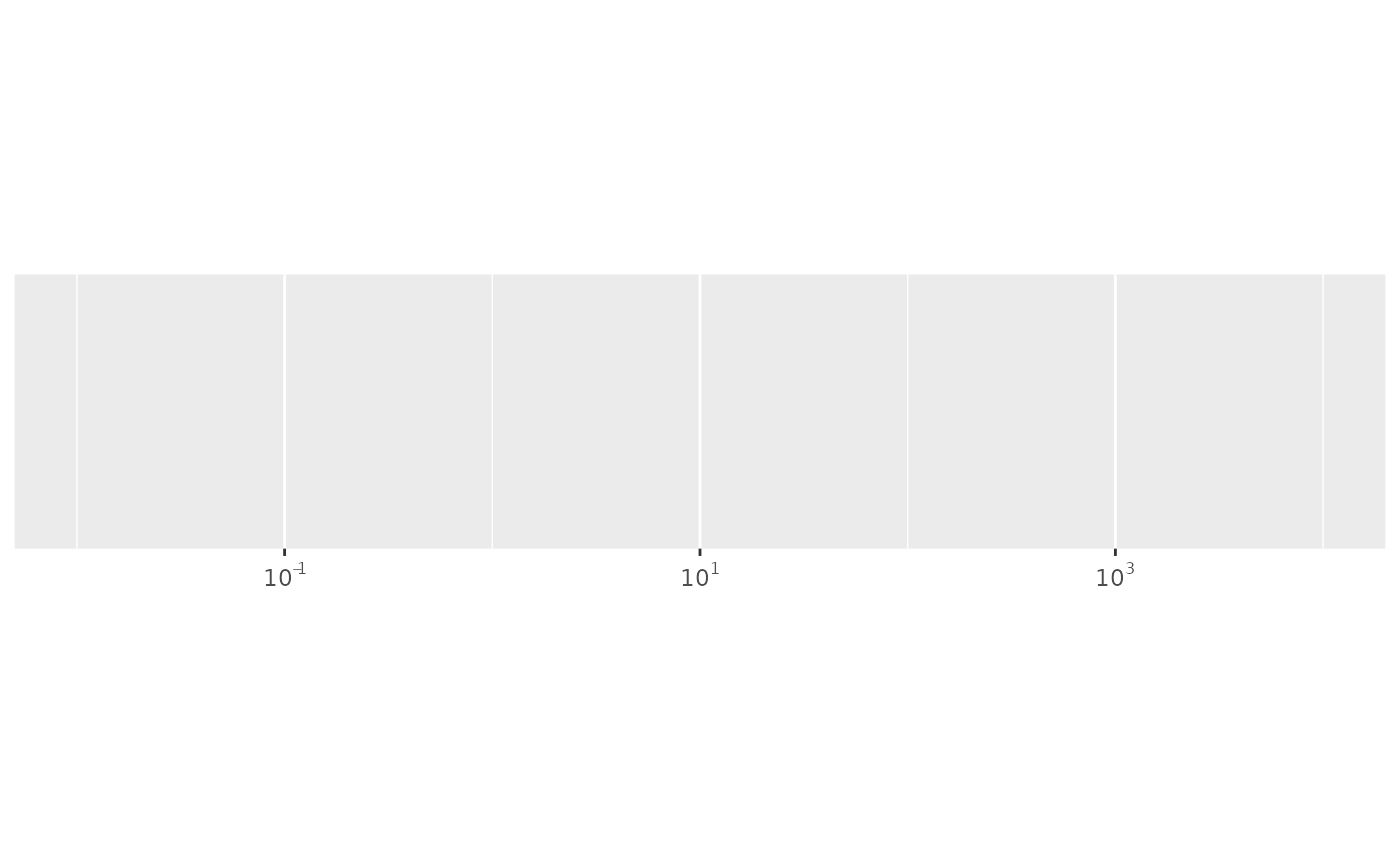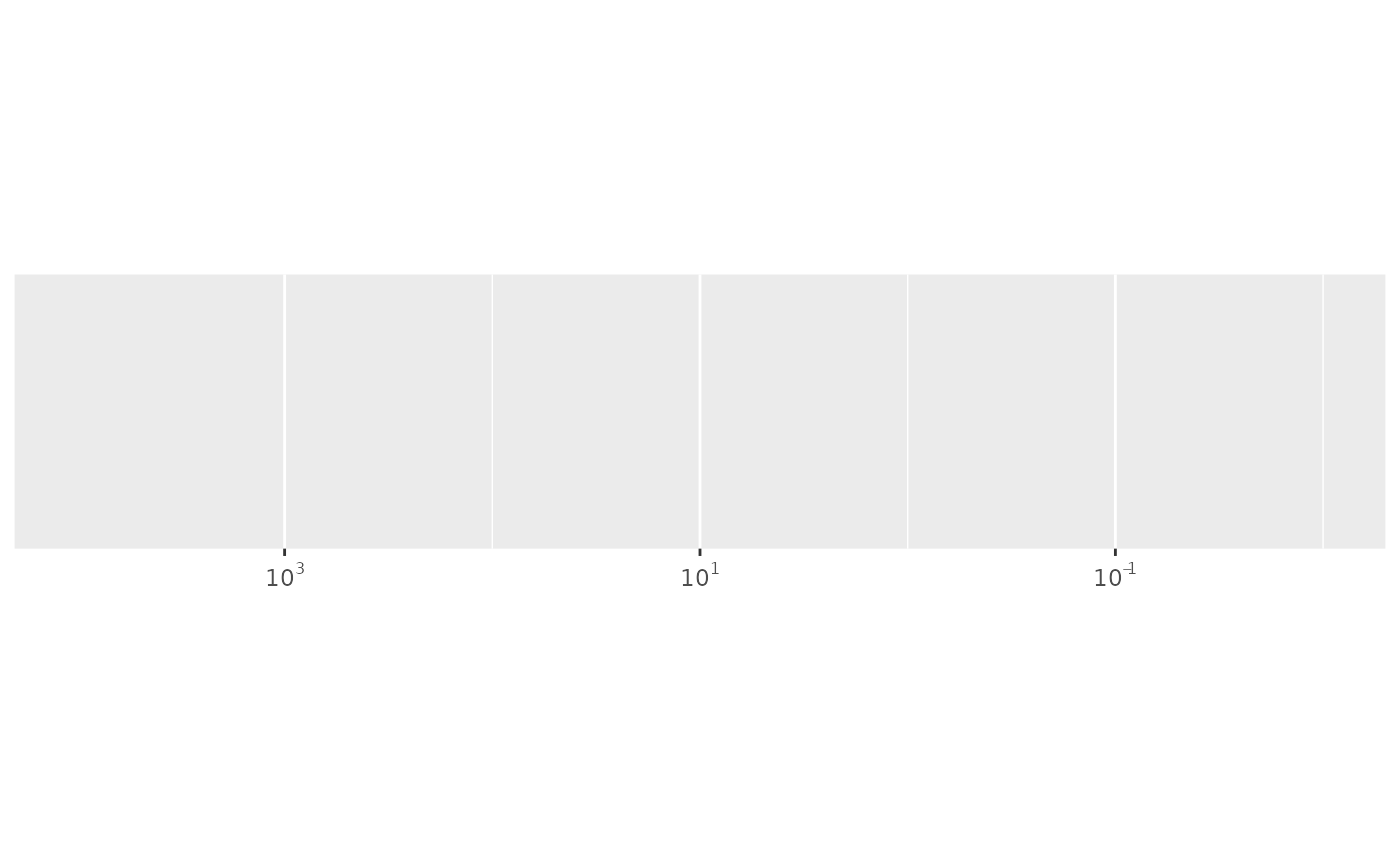This transformer provides a general mechanism for composing two or more transformers together. The most important use case is to combine reverse with other transformations.

## Usage

compose_trans(...)

## Arguments

...

One or more transformers, either specified with string or as individual transformer objects.

## Examples

demo_continuous(10^c(-2:4), trans = "log10", labels = label_log())
#> scale_x_continuous(trans = "log10", labels = label_log())demo_continuous(10^c(-2:4), trans = c("log10", "reverse"), labels = label_log())
#> scale_x_continuous(trans = c("log10", "reverse"), labels = label_log())